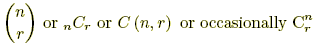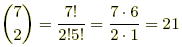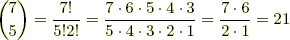index: click on a letter A B C D E F G H I J K L M N O P Q R S T U V W X Y Z A to Z index index: subject areas numbers & symbols sets, logic, proofs geometry algebra trigonometry advanced algebra & pre-calculus calculus advanced topics probability & statistics real world applications multimedia entrieswww.mathwords.com about mathwords website feedback

Binomial Coefficients

Numbers written in any of the ways shown below. Each notation is read aloud "n choose r."A binomial coefficient equals the number of combinations of r items that can be selected from a set of n items. It also represents an entry in Pascal's triangle. These numbers are called binomial coefficients because they are coefficients in the binomial theorem.

 Formula:Note:, where nPr is the formula for permutations of n objects taken r at a time. Examples: